この節の作者: Jonathon Love

In jamovi, data is represented in a spreadsheet, with each column representing a “variable”.

## Data Variables¶

The most commonly used variables in jamovi are “Data Variables”, these variables simply contain data either loaded from a data file, or “typed in” by the user. Data variables can be one three data types:

• `Integer`
• `Decimal`
• `Text`

and one of four measure types:

The measure types are designated by the symbol in the header of the variable’s column. Note that some combinations of data-type and measure-type don’t make sense, and jamovi won’t let you choose these.

`Nominal` and `Ordinal` are, predictably, for nominal and ordinal variables. `Continuous` is for variables with numeric values which are considered to be Interval or Ratio scales (Equivalent to `Scale` in SPSS). The `ID` measure type is, unlike the others, unique to jamovi. It’s intended for variables that contain identifiers that you would almost never want to analyse. For example, a persons name, or a participant ID. The advantage of IDs, is that jamovi does not need to maintain a list of levels internally, which can improve performance when interacting with very large data sets.

When starting with a blank spreadsheet and typing values in, the data and measure types will change automatically depending on the data you enter. This is a good way to get a feel for which variable types go with which sorts of data. Similarly, when opening a data file, jamovi will infer the variable type from the data in each column. In both cases, this automatic approach may not be correct, and it may be necessary to manually specify the data and measure type with the variable editor.

The variable editor can be invoked by selecting `Setup` from the `Data` tab, double-clicking on the column header, or by pressing `F3`. The variable editor allows you to change the name of the variable, and (for data variables) the data type, the measure type, the order of the levels, and the label displayed for each level. The variable editor can be dismissed by clicking the close arrow, or by pressing `F3` again.

New variables can be inserted or appended to the data set using the `Add` button from the data ribbon. The `Add` button also allows the addition of Computed variables.

## Computed Variables¶

Computed Variables are those which take their value by performing a computation on other Variables. Computed Variables can be used for a range of purposes, including log transforms, z-scores, sum-scores, negative scoring and means.

Computed variables can be added to the data set, with the `Add` button available on the data tab. This will produce a formula box where you can specify the formula. The usual arithmetic operators are available. Some examples of formulas are:

```A + B
LOG10(len)
MEAN(A, B)
(dose - VMEAN(dose)) / VSTDEV(dose)
Z(dose)
```

In order, these are the sum of A and B, a log (base 10) transform of `len`, the mean of `A` and `B`, and the z-score of `dose` (twice).

There are many more functions available.

### `V`-functions¶

A number of functions appear in pairs, one prefixed with a `V` and the other not. `V` functions perform their calculation on a variable as a whole, where as non-`V` functions perform their calculation row by row. For example, `MEAN(A, B)` will produce the mean of `A` and `B` for each row. Whereas `VMEAN(A)` gives the mean of all the values in `A`.

Additionally `V` functions support a `group_by` argument. When a `group_by` variable is specified, a separate value is calculated for each level of the `group_by` variable. In the following example:

```VMEAN(len, group_by=dose)
```

A separate mean is calculated for each level of `dose`, and each value in the computed variable will be the mean corresponding to it’s row’s value of `dose`.

## Transformed (Recoded) Variables¶

While computed variables are great for a lot of operations (e.g., calculating sum scores, generating data, etc.), they can be a bit tedious to use when you want to recode or transform multiple variables (e.g., when reverse-scoring multiple responses in a survey data set). “Transformed variables” allow you to easily recode existing variables and apply transforms across many variables at once.

Creating transformed variables

When transforming or recoding variables in jamovi, a second “transformed variable” is created for the original “source variable”. This way, you will always have access to the original, untransformed data if need be. To transform a variable, first select the column(s) you would like to transform. You can select a block of columns by clicking on the first column header in the block and then clicking on the last column header in the block while holding the shift key. Alternatively, you can select / deselect individual columns by clicking on the column headers while holding down the Ctrl / Cmd key. Once selected, you can either select `Transform` from the data tab, or right click and choose `Transform` from the menu.

Either right-click on one of the selected variables, and click `Transform...`: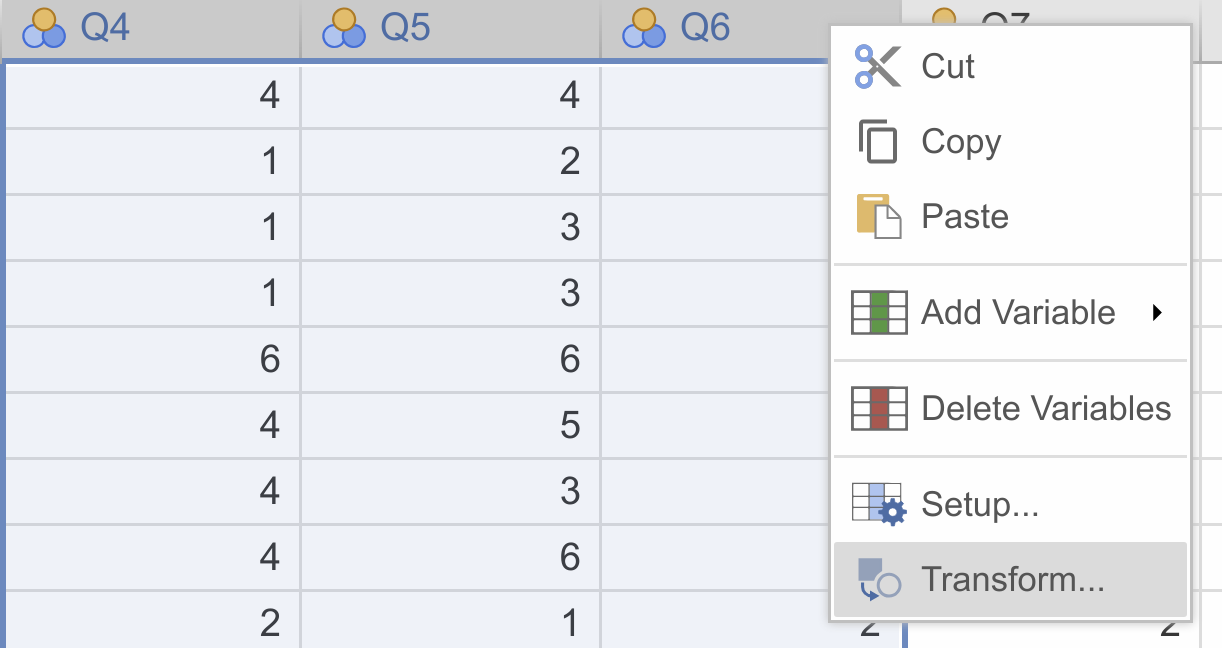or head to the `Data`-ribbon, and click `Transform:`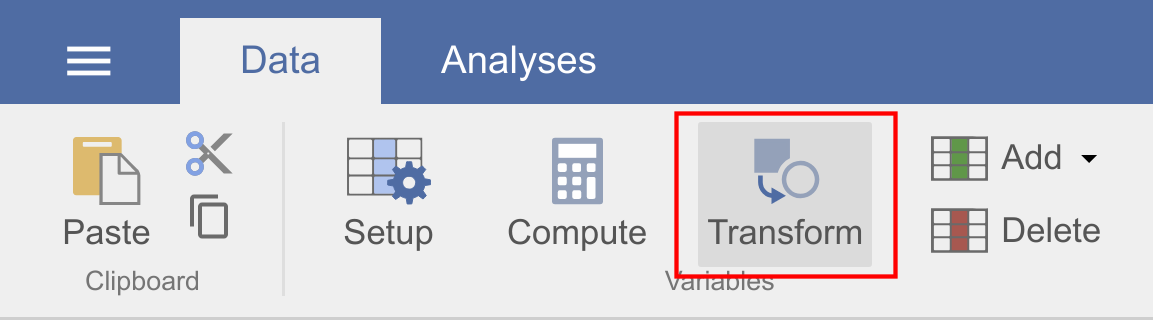This constructs a second “transformed variable” for each column that was selected. In the following example, we only had a single variable selected, so we’re only setting up the transform for one variable (called score - log), but there’s no reason we can’t do more in one go.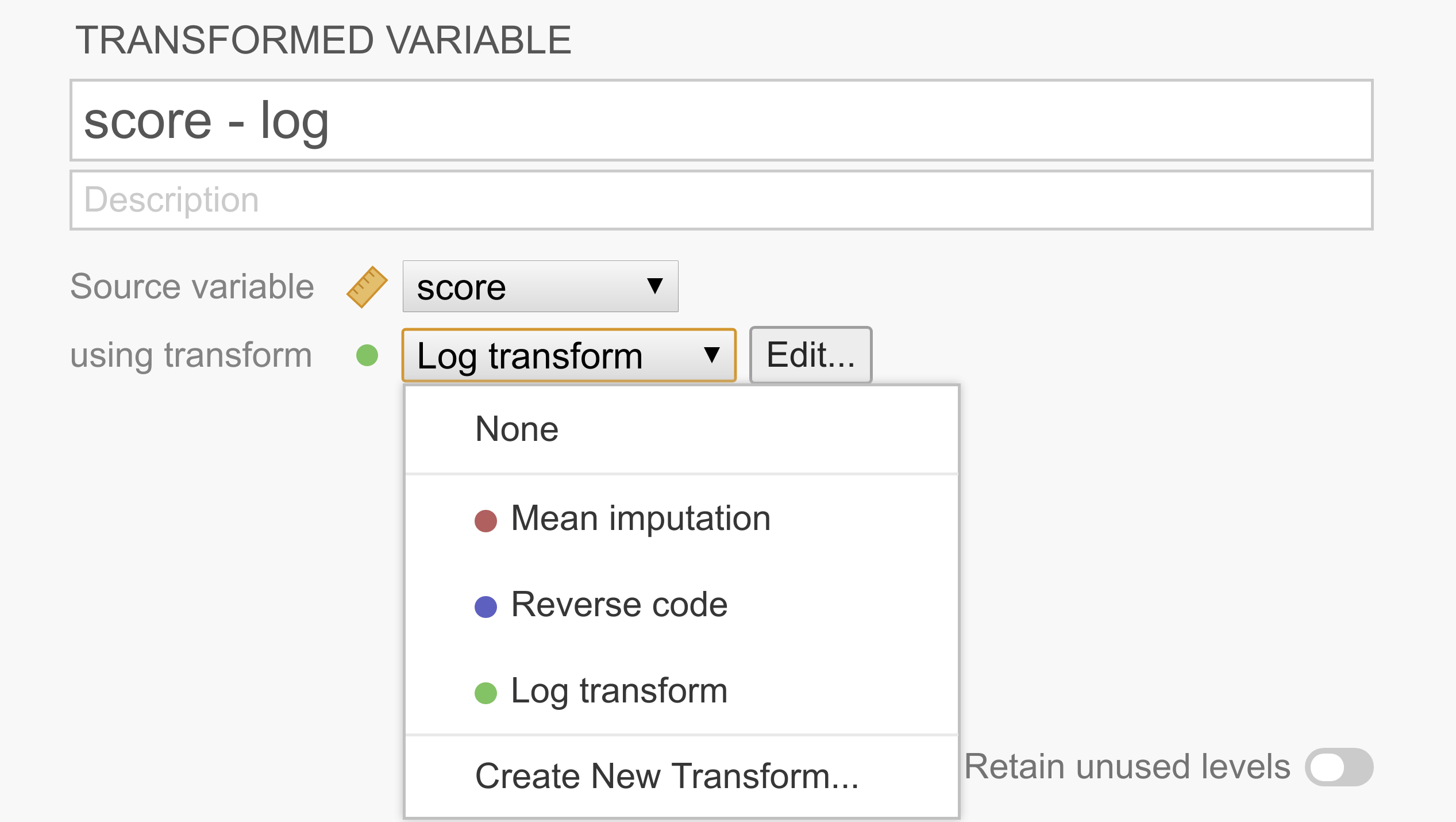As can be seen in the figure above, each transformed variable has a “source variable”, representing the original untransformed variable, and a transform, representing rules to transform the source variable into the transformed variable. After a transform has been created, it’s available from the list and can be shared easily across multiple transformed variables.

If you don’t yet have the appropriate transform defined, you can select `Create new transform...` from the list.

Create a new transformation

After clicking `Create new transform...` the transform editor slides into view: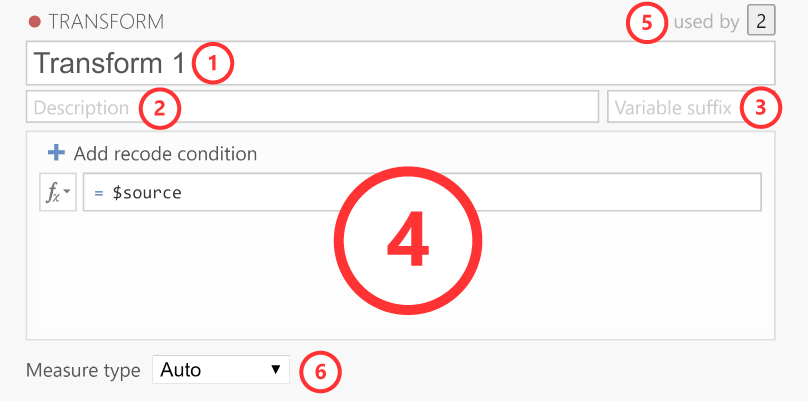The transform editor contains these elements.

1. Name: The name for the transformation.

2. Description: Space for you to provide a description of the transformation so you (and others) know what it does.

3. Variable suffix (optional): Here, you can define the default name formatting for the transformed variable. By default, the variable suffix will be appended to the source variable name with a dash (-) in between. However, you can override this behavior by using an three dots (...), which will be replaced by the variable name. For instance, if you transform a variable called Q1, you could use variable suffixes to apply the following naming schemes (if left empty, the transformation name is used as the variable suffix):

`log``Q1 - log` `..._log``Q1_log` `log(...)``log(Q1)`

4. Transformation: This section contains the rules and formulas for the transformation. You can use all the same functions that are available in computed variables, and to refer to the values in the source column (so you can transform them), you can use the special `\$source` keyword. If you want to recode a variable into multiple groups, it’s easiest to use multiple conditions. To add additional conditions (i.e., if-statements), you click on the `Add recode condition` button:

5. Used by: Indicates how many variables are using this particular transformation. If you click on the number it will list these variables.

6. Measure type: By default the measure type is set to Auto, which will infer the measure type automatically from the transformation. However, if Auto doesn’t infer the measure type correctly, you can override it over here.

Example 1: Reverse scoring of items

Survey data often contains one or more items whose values need to be reversed before analyzing them. For example, we might be measuring extraversion with the questions “I like to go to parties”, “I love being around people”, and “I prefer to keep to myself”. Clearly a person responding 6 (strongly agree) to this last question shouldn’t be considered an extravert, and so 6 should be treated as 1, 5 as 2, 1 as 6, etc. To reverse score these items, we can just use the following simple transform: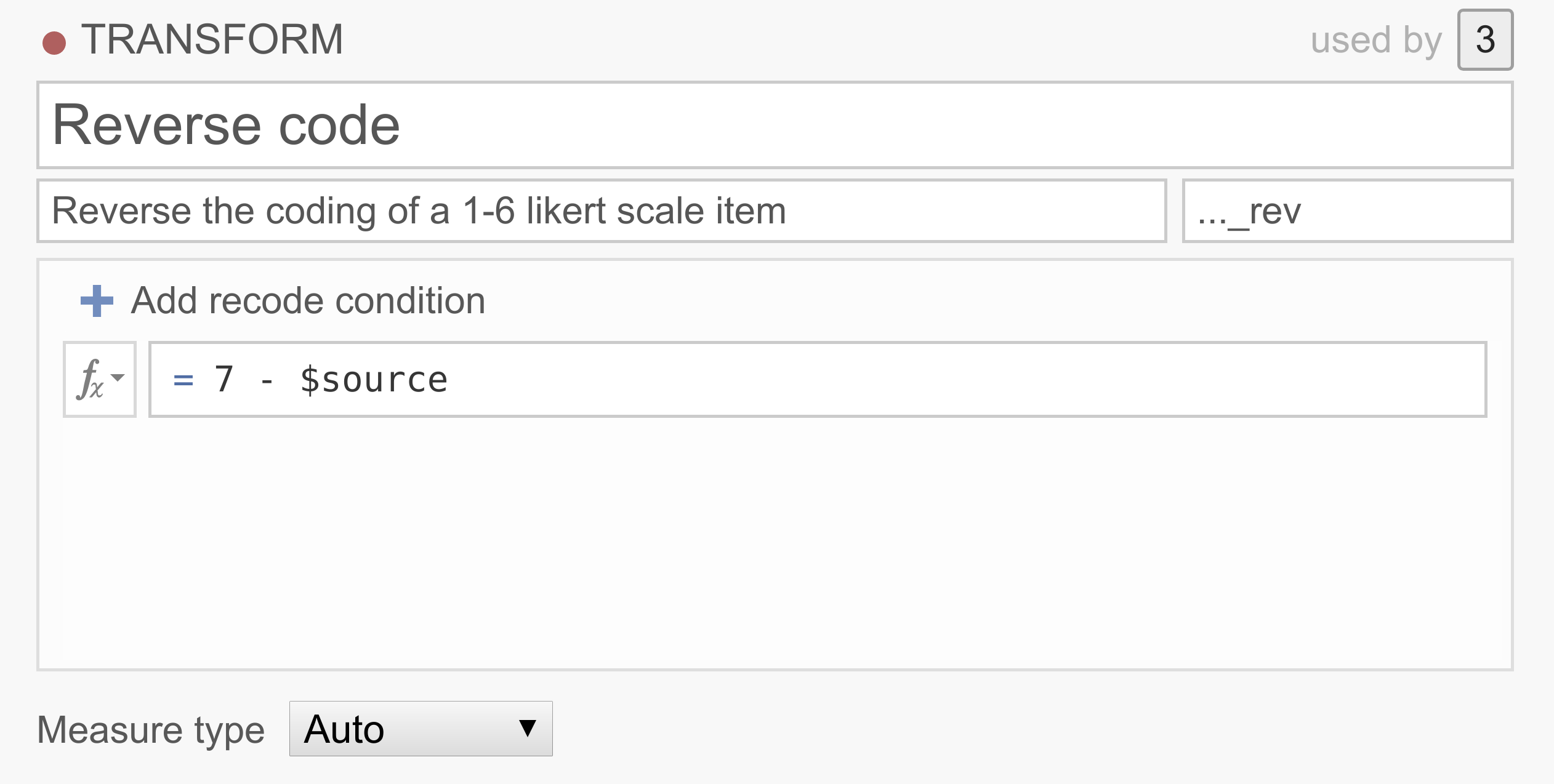You can explore this transform by downloading <../_static/output/um_transform_ex1.omv> and opening the file `transform_ex1` in jamovi.

Example 2: Recoding continuous variables into categories

In a lot of data sets people want to recode their continuous scores into categories. For example, we may want to classify people, based on their 0-100% test scores into one of three groups, `Pass`, `Resit` and `Fail`.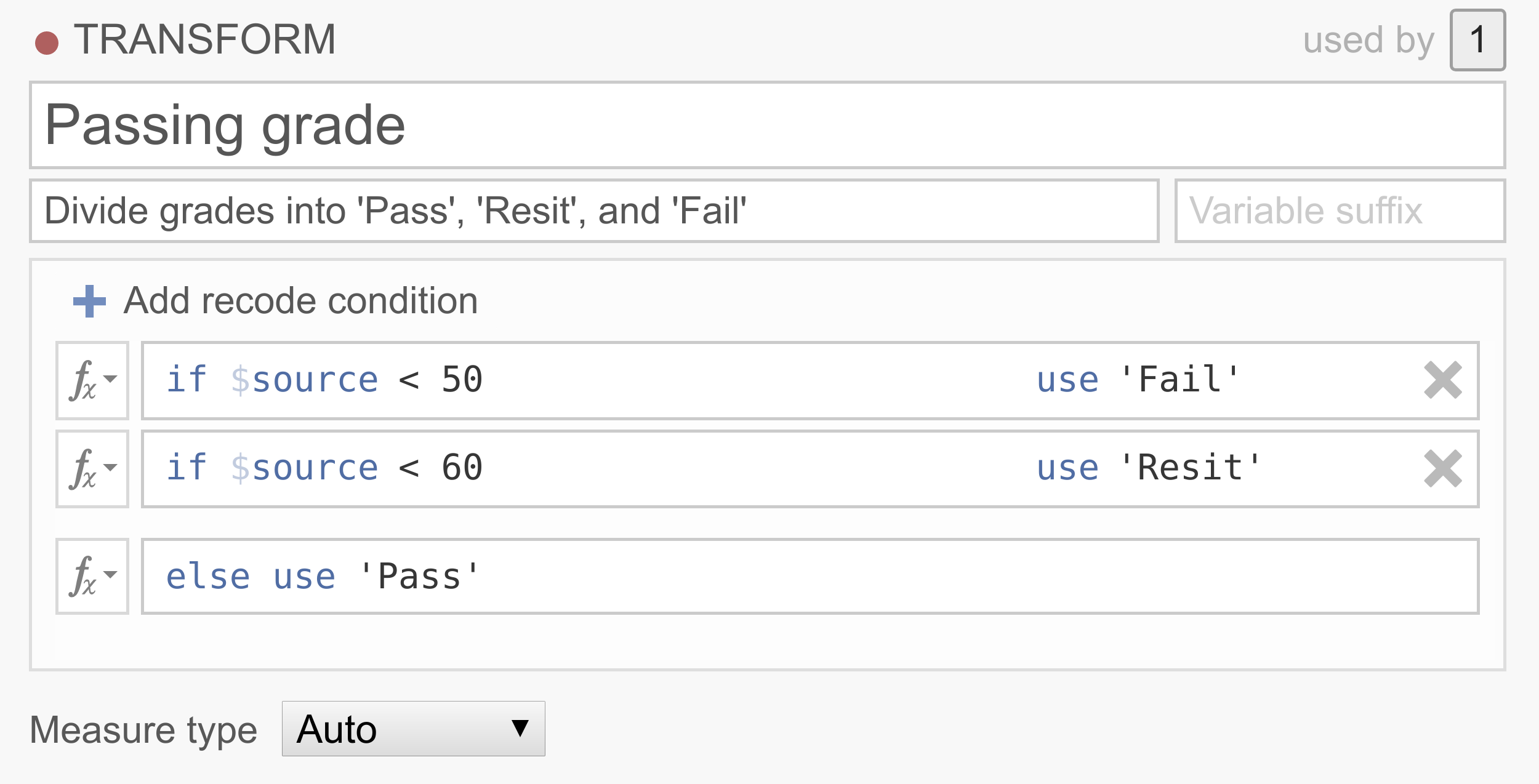Note that the conditions are executed in order, and that only the first rule that matches the case is applied to that case. So this transformation basically says that if the source variable has a value below 50, the value will be `Fail`, if the source variable has a value between 50 and 60, the value will be `Resit`, and if the source variable has a value above 60, the value will be `Pass`. If you’d like an example data set to play around with, you can download <../_static/output/um_transform_ex2.omv> and use `transform_ex2.omv`.

Example 3: Replacing missing values

Now, let’s say your data set has a lot of missing values and removing the participants with missing values will end up in a severe loss of participants. There are a number of ways to deal with missing data, of which imputation is quite common. One pretty straightforward imputation method replaces the missing values with the variable mean (i.e., mean substitution). Although there are a bunch of problems associated with mean substitution and you should probably never do it, it does make for a neat demonstration...Note that jamovi has borrowed NA from R to denote missing values. Don’t have a good data set handy? You can try it out yourself by downloading <../_static/output/um_transform_ex3.omv> and opening the `transform_ex3.omv` data set.

## Filters¶

Filters in jamovi allow you to filter out rows that you don’t want included in your analysis. For example, you might want to only include people’s survey responses if they explicitly consented to having their data used, or you might want to exclude all left-handed people, or perhaps people who score “below chance” in an experimental task. In some cases you just want to exclude extreme scores, for example those that score more than 3 standard deviations from the mean.

The filters in jamovi are build on top of jamovi’s computed variable formula system, which allows the building of arbitrarily complex formulas.

Row filters

jamovi filters are demonstrated using the `Tooth Growth` data set which is included with jamovi (`☰``Open``Data Library`). Select the `Filters button` from the `Data` ribbon. This opens the filter view and creates a new filter called `Filter 1`.

In the short video, we specify a filter to exclude the 9th row. Perhaps we know that the 9th participant was someone just testing the survey system, and not a proper participant (`Tooth Growth` is actually about the length of guinea pig teeth, so perhaps we know that the 9th participant was a rabbit). We can simply exclude them with the formula:

```ROW() != 9
```

In this expression the `!=` means ‘does not equal’. If you’ve ever used a programming language like R this should be very familiar. Filters in jamovi exclude the rows for which the formula is not true. in this case, the expression `ROW() != 9` is true for all rows except the 9th row. When we apply this filter, the tick in the `Filter 1` column of the 9th row changes to a cross, and the whole row greys out. If we were to run an analysis now, it would run as though the 9th row wasn’t there. Similarly, if we already had run some analyses, they would re-run and the results would update to values not using the 9th row.

Typically, we would like to have more complex filters than this! The ```Tooth Growth``` example contains the length of teeth from guinea pigs (the `len` column) fed different dosages (the `dose` column) of supplements: vitamin C or orange juice (recorded in the `supp` column as `VC` and `OJ`). Let’s assume that we’re interested in the effect of dosage on tooth length. We might run an ANOVA with `len` as the dependent variable, and `dose` as the grouping variable. But let’s say that we’re only interested in the effects of vitamin C, and not of orange juice. Then, we can use the formula:

```supp == 'VC'
```

In fact we can specify this formula in addition to the `ROW() != 9` formula if we like. We can add it as another expression to `Filter 1` (by clicking the small `+` beside the first formula), or we can add it as an additional filter (by selecting the large `+` to the left of the filters dialog box). As we’ll see, adding an expression to an existing filter does not provide exactly the same behaviour as creating a separate filter. In this case however, it doesn’t make a difference, so we’ll just add it to the existing filter. This additional expression comes to be represented with its own column as well, and by looking at the ticks and crosses, we can see which filter or expression is responsible for excluding each row.

But let’s say we want to exclude from the analysis all the tooth lengths that were more than 1.5 standard deviations from the mean. To do this, we’d take a z-score, and check that it falls between -1.5 and 1.5. we could use one of the following formulas (this second formula is a great way to demonstrate to students what a z-score is):

```-1.5 < Z(len) < 1.5
-1.5 < (len - VMEAN(len)) / VSTDEV(len) < 1.5
```

There are a lot of functions available in jamovi, and you can see them by clicking the small fx beside the formula box.

Now let’s add this z-score formula to a separate filter by clicking the large `+` to the left of the filters, and adding it to `Filter 2`.

With multiple filters, the filtered rows cascade from one filter into the next. So only the rows allowed through by `Filter 1` are used in the calculations for `Filter 2`. In this case, the mean and standard deviation for the z-score will be based only on the vitamin C rows (and also not on row 9). In contrast, if we’d specified our `Z()` filter as an additional expression in `Filter 1`, then the mean and standard deviation for the z-score would be based on the entire dataset. In this way you can specify arbitrarily complex rules for when a row should be included in analyses or not (but you should pre-register your rules).

Column filters

Whereas row filters are applied to the data set as a whole, sometimes you want to just filter individual columns. Column filters come in handy when you want to filter some rows for some analyses, but not for all. This is achieved with the computed variable system. With the computed variables we create a copy of an existing column, but with the unwanted values excluded.

In the Tooth Growth example, we might want to analyse the doses of 500 and 1000, and 1000 and 2000 separately. To do this we create a new column for each subset. So in our example, we can select the dose column in the jamovi spreadsheet, and then select the Compute button from the data tab. This creates a new column to the right called dose (2), and same as the filters, we can enter a formula. in this case we’ll enter one of the formulas below (the do the same, the second is perhaps easier to understand):

```FILTER(dose, dose <= 1000)
FILTER(dose, dose == 1000 or dose == 500)
```

The first argument to the `FILTER()` function (in this example dose) is what values to use in the computed column. The second argument is the condition; when this condition isn’t satisfied, the value comes across blank (or as a “missing value” if you prefer). So with this formula, the `dose (2)` column contains all the `500` and `1000` values, but the `2000` values are not there.

We might also change the name of the column to something more descriptive, like `dose 5,10`. Similarly we can create a column `dose 10,20` with the formula `FILTER(dose, dose != 500)`. Now we can run two separate ANOVAs (or t-tests) using `len` as the dependent variable, and `dose 5,10` as one grouping variable in the first analysis, and `dose 10,20` in the other. In this way we can use different filters for different analyses. Contrast this with row filters which are applied to all the analyses.

It may also have occurred to you, that with `FILTER()` we can do what might be called a “poor man’s split variables”: You can create splits using `FILTER()`. For example, we could split `len` into two new columns `len_VC` and `len_OJ` with the functions `FILTER(len, supp == 'VC')` and `FILTER(len, supp == 'OJ')` respectively. This results in two separate columns which can be analysed side-by-side.

  Pre-registration is a solution to p-hacking, not deliberately making software difficult to use! Don’t p-hacking. Your p-hacking harms more people than you may assume.